# Cumulate IssueHi All,

I just need a little opinion whether excel is wrong or Anaplan. See below.

So i only copied the values so there is no formula.

Excel Driver Cumulate formula it adds the Excel Source value and Excel Source Plus value. So its like Sum(Value, PLus Value).

The problem that I have is in Excel Driver when they cumulate Month 1 and Month 2 are both 25.

In Anaplan, Month 1 is 25 and Month 2 is 38 (25+13). Which one is correct the excel or Anaplan formula? I just need to get some insights on this to understand better where my formula encountered a problem.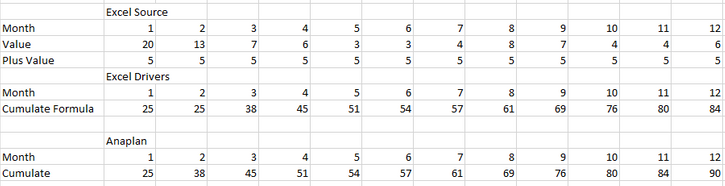Appreciate  the response.

Regards

Tagged:

•Neat use case!

My initial reaction to this is that you shouldn't use CUMULATE.

Rather, try using PREVIOUS.

So your formula would be cumualte value = PREVIOUS(cumulate Value) + Value + Add Value.

PREVIOUS function in Anaplan is much more efficient over short time periods than CUMULATE since CUMULATE has to recalculate every single time period across the entire time frame.

Try that. If you want a screenshot or two, I can provide you one showing you how to get these numbers.

•Hi, @JaredDolich

I don't think this is so automatic. We have to make another line item to use the PREVIOUS() function instead of CUMULATE().
CUMULATE(Value) + plus Value <> PREVIOUS(Cumulate) + Value + plus Value.
In this case, PREVIOUS() running sums plus Value together. That is not the desired outcome.

INCORRECT BELOW. I have to reconsider..

Now I don't clearly understand what "Excel Driver" means. If I reproduce this behavior of the function with Anaplan calculation, it may be like this.
new LINE ITEM = OFFSET(Cumulate Formula, -2, 0) + OFFSET(Value, -1, Value)
Cumulate Formula = new LINE ITEM + plus Value

I cannot understand why so odd way this function runs.
It may be just my misunderstanding about how it works.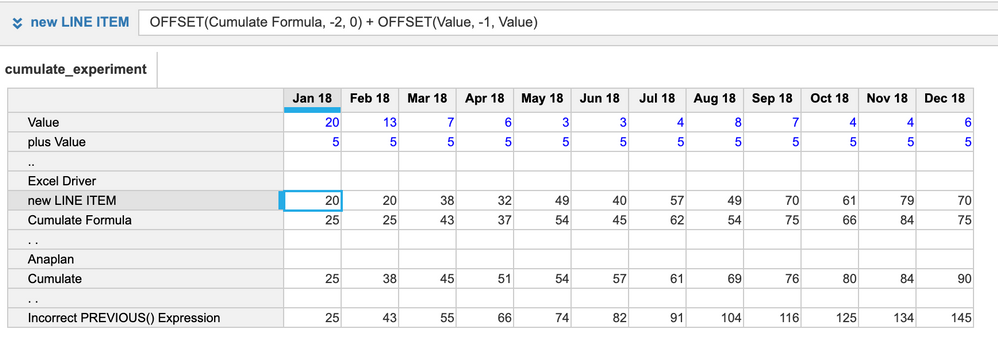Regards,

Taichi

•Can you update the excel formula and the anaplan formula that you are comparing.

Thanks

Arun

•Hopefully, I've understood the use case correctly.

So here's an example using your numbers. I didn't have to add any extra line items.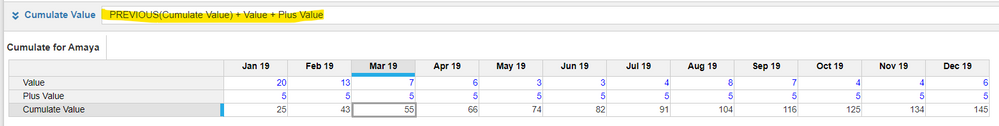And the solution for Excel is the same.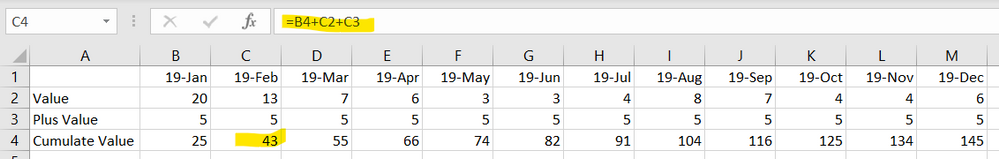If this isn't giving you the numbers you expected, can you share what the right values should be for the cumulate value?

•Thank you, @JaredDolich

I apologize to make a topic confused.

Please take a look at the screenshot on top of this topic, the Cumulate on the 12th month is 90. And yours is 145. That's the difference between cumulate and previous, I think.

But this may go off a topic. Actually, I am not so sure what this discussion is about.
It would be better for me to wait for some further information.
•Its seem the formula which you have written for excel driver is not correct. I tried to replicate the same calculation both in Excel as well as in Anaplan and I got the same result.

Excel: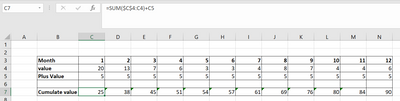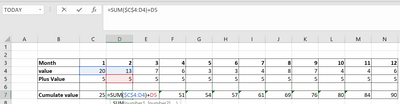Anaplan: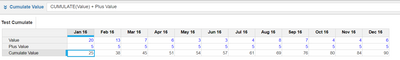Note:

Cumulate is faster for short Time Period and for long timescales, using PREVIOUS is faster than CUMULATE due to the number of "reads" required for the calculation.

Hope this help!

Thanks

Akhtar

•Hi Jared, Thank you for taking time in answering my query. Regards,